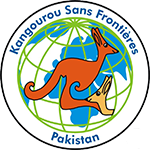## Kangaroo-Ecolier Test Preparation

### Unlimited Printable Worksheets, Online Practice and Online Tests For Kangaroo-Ecolier• The duration of the competition is 2 hours.
• 24 problems to solve: eight - 3 points each, eight - 4 points each and eight - 5 points each.
• Calculators are not allowed. You may use basic geometric tools.
• Scope of problems:
• simple arithmetic operations with 1,2,3 and 4 digit numbers.
• recognizing geometric figures.
• a magic square with a sum of 15.
• time, clock.
• number of days in a week, number of months in a year.
• intersection of sets.
• perimeter and area of a square, a rectangle.
(Source : http://ikmc.kangaroo.org.pk/ )
Mixed Operations, Logical Reasoning, Time, Shapes and Patterns, Large Numbers, Geometry, Multiplication, Roman Numerals, Division, Fractions, Measurement - Length, Mass & Capacity, Natural and Whole Numbers, . . .

Large Numbers, Roman Numerals, Multiplication, Division, Mixed Operations, Unitary Method, Geometry, Natural and Whole Numbers, Fractions, Measurement - Length, Mass & Capacity, Decimals, Time, Mensuration - Perimeter, Area, Volume, etc., . . .